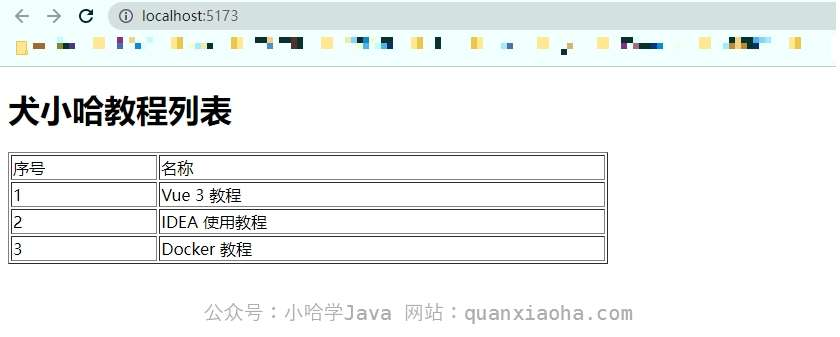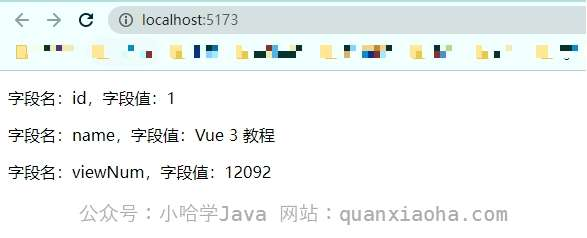# Vue 3 循环遍历（超详细）

## v-for 指令基础用法

### 第一种方式

``````v-for="item in list"
``````
• `item` 表示当前正在遍历的对象；
• `in` 是固定语法，表示对 `list` 数组进行遍历；
• `list` 表示被遍历的数组。

### 第二种方式

``````v-for="(item, index) in list" :key="index"
``````

### 使用示例

``````<template>
<h1>犬小哈教程列表</h1>
<table border="1" width="600px">
<tr>
<td>序号</td>
<td>名称</td>
</tr>
<tr v-for="(tutorial, index) in tutorials" :key="index">
<td>{{ index + 1 }}</td>
<td>{{ tutorial }}</td>
</tr>
</table>
</template>

<script setup>
import { ref } from 'vue'

// 定义一个教程列表数组
const tutorials = ref(['Vue 3 教程', 'IDEA 使用教程', 'Docker 教程'])
</script>
``````v-for 指令遍历基本用法

## 遍历对象数组

``````<template>
<h1>犬小哈教程列表</h1>
<table border="1" width="600px">
<tr>
<td>ID</td>
<td>名称</td>
<td>阅读量</td>
</tr>
<tr v-for="(tutorial, index) in tutorials" :key="index">
<td>{{ tutorial.id }}</td>
<td>{{ tutorial.name }}</td>
<td>{{ tutorial.viewNum }}</td>
</tr>
</table>
</template>

<script setup>
import { reactive } from 'vue'

// 定义一个教程列表数组, 元素为对象，包含 ID、教程名称、被阅读数 3 个字段
const tutorials = reactive([
{id: 1, name: "Vue 3 教程", viewNum: "12092"},
{id: 2, name: "IDEA 教程", viewNum: "90236"},
{id: 3, name: "Docker 教程", viewNum: "5902"},
])
</script>
``````v-for 指令遍历对象数组

## 遍历对象

``````v-for="(value, key, index) in Object" :key="index"
``````
• `Object` 表示被遍历的对象；
• `key` 表示对象的字段名；
• `value` 表示对象的字段值；
• `index` 表示当前遍历的索引下标。

``````<template>
<p v-for="(value, key, index) in tutorial" :key="index">
字段名：{{ key }}，字段值：{{ value }}
</p>
</template>

<script setup>
import { reactive } from 'vue'

// 定义一个对象，包含 ID、教程名称、被阅读数 3 个字段
const tutorial = reactive({id: 1, name: "Vue 3 教程", viewNum: "12092"},)
</script>
``````v-for 指令遍历对象